## Section 1. Introduction to finite mixture models and mixComp

Mixture models have been used extensively in statistical applications and therefore have attracted a lot of attention from both theoretical and computational perspectives. Although the list of works on mixture models is too long to make an exhaustive inventory, we can refer to the following important papers and books: [@Teicher63], [@LindsayI], [@LindsayII], [@Titterington] and [@McLachlan].

The popularity of such models stems from the fact that they allow for modeling heterogeneous data whose distribution cannot be captured by a single parametric distribution. To account for such heterogeneity, the (unknown) distribution is assumed to result from mixing over some latent parameter in the following sense: the latent parameter is viewed itself as a random variable drawn from some unknown mixing distribution. When this mixing distribution is only assumed to belong to the ensemble of all possible distribution functions, the mixture model is called \textit{nonparametric} and estimation of the mixing distribution requires using some nonparametric estimation method. This includes the well-known nonparametric maximum likelihood estimator (NPMLE) whose fundamental properties were well studied in the seminal work of [@LindsayI], [@LindsayII]. One remarkable property of the NPMLE of the mixing distribution is that it is, under some simple conditions, a discrete distribution function with at most $$k$$ number of jumps, where $$k$$ is the number of distinct observations in the random sample drawn from the mixture. This interesting feature is one reason, among others, for considering the smaller class of finite mixture models, i.e., mixture models with a discrete mixing distribution with a finite number of jumps. The model has the following simple interpretation: the population under study is assumed to consist of several homogeneous subpopulations. These subpopulations, typically referred to as the mixture's components, usually have a specific meaning depending on the problem at hand. In some very simple situations, the number of components could be known in advance, in which case the model is \textit{fully parametric} and convergence of classical estimators such as the parametric maximum likelihood estimator (MLE) is known to occur at the fast rate $$\sqrt{n}$$ (under some regularity conditions). Also, the well-known expectation-maximization (EM) algorithm can be used to find the MLE of all the unknown parameters; see for example [@Dempster]. However, in many statistical applications such knowledge is rarely available and the number of components has to be estimated from the data. Although the mixture is still finite and the distribution of each component is assumed to belong to some parametric family, the estimation framework in this case is much harder than in the fully parametric one, where the number of components is known. In this paper, the terms \textit{order}, \textit{complexity} and \textit{number of components} will be used interchangeably to refer to this unknown number. The main goal of the package \pkg{mixComp} is to estimate the unknown complexity using several methods known from the statistical literature. These methods, which are discussed below in more detail, all come with theoretical guarantees for consistency as the sample size gets larger. Of course, consistency in this case means that an estimator is able to exactly recover the unknown complexity for large sample sizes. As expected, the performance of the methods varies according to the underlying mixture distribution and the sample size. This will be illustrated below through several synthetic as well as real datasets.

To describe the estimation problem, we start with some formal notation. A distribution $$F$$ is called a \textit{finite mixture} if its density (we write density throughout and keep in mind that it may be taken with respect to the Lebesgue or the counting measure) is of the form

$f(x) = \sum_{i=1}^p w_i g_i(x),$

where $$p \in \mathbb{N}$$ is the mixture complexity, $$(w_1, \dots w_p : \sum_{i=1}^p w_i = 1$$, $$w_i \geq 0,$$ for $$i=1,\dots,p)$$ are the component weights and the density $$g_i(x)$$ is the $i$th component of the mixture. As the scope of \pkg{mixComp} is limited to mixtures where the family of the component distributions is known, we replace $$g_i(x)$$ by a parametric density $$g(x; \theta_i)$$ indexed by the (possibly multivariate, say $$d$$-dimensional) parameter $$\theta_i$$ in the parameter space $$\Theta \subseteq \mathbb{R}^d$$.

Given some complexity $$j$$, the two relevant parameter spaces can therefore be defined as $\Theta_j = \{\theta_1 \dots \theta_j: \theta_i \in \Theta \subseteq \mathbb{R}^d, \text{ for } i = 1,\dots,j\}$ and $W_j = \{w_1, \dots, w_j: \sum_{i=1}^j w_i = 1, w_i \geq 0, \text{ for } i = 1,\dots,j\}.$

Throughout this document, it is assumed that the family of the component densities $$\{g(x; \theta):\theta \in \Theta\}$$ is known, but the component parameters $$\mathbf{\theta} = (\theta_1, \dots, \theta_p) \in \Theta_p$$, the component weights $$\textbf{w} = (w_1, \dots, w_p) \in W_p$$ and the mixture complexity $$p \in \mathbb{N}$$ are unknown, with $$p$$ being the parameter of interest. Assume now that $$F$$ is a finite mixture distribution with density $f(x) = \sum_{i=1}^p w_i g(x; \theta_i)$ and $$\textbf{X} = \{X_1, \dots, X_n\}$$ is an i.i.d. sample of size $$n$$ from $$F$$. The mixComp package aims to estimate the smallest such $$p$$ on the basis of $$\textbf{X}$$, either on its own or by simultaneously estimating the weights $$w_i$$ and the component parameters $$\theta_i$$, $$i \in 1, \dots, p$$.

In this setup, it seems natural to test for the number of components by comparing two consecutive models (with $$j$$ and $$j+1$$ components, say) and regarding the mixture with $$j$$ components as the imposition of a null hypothesis on the larger model (intuitively, the null hypothesis $H_0: \left\{ \exists i \in \{1, 2, \dots, j+1\}: w_{i} = 0 \right\}$ comes to mind). Traditionally, the problem of testing between nested models may be approached by applying the generalized likelihood ratio test and referring to the $$\chi^2_r$$ distribution to assess significance, where $$r$$ is given by the number of constraints imposed on $$H_1$$ in order to arrive at $$H_0$$. However, in the context of mixture models, there is no unique way of obtaining $$H_0$$ from $$H_1$$. As an example, the two null hypotheses $$H_0: w_{j+1} = 0$$ and $$H_0: \theta_{j+1} = \theta_{1}$$ both yield the smaller model, demonstrating the difficulties of applying this method. This problem has been studied extensively in the literature and numerous other approaches to complexity estimation have been suggested, laying the theoretical foundation for the algorithms described further.

This document discusses various categories of functions found in the mixComp package, ranging from methods based on Hankel matrices, to techniques based upon distances between densities and likelihood ratio tests. The examples provided in the first sections all contain mixtures of “standard” distributions for which evaluation of the density, cumulative distribution function and quantile function as well as random variate generation may be performed using the functions from the stats package. The last section illustrates how the mixComp package can be used to estimate the complexity of any mixture as long as the user provides functions generating random variates from the component distribution and valuating the density thereof.

Two main features distinguish this package from other mixture-related R [@R] packages: Firstly, it is focused on the estimation of the complexity rather than the component weights and parameters. While these are often estimated as a by-product, all methods contained in mixComp are based on theory specifically developed to consistently estimate the number of components in the mixture of interest. Secondly, it is applicable to parametric mixtures well beyond those whose component distributions are included in the stats package, making it more customizable than most packages for model-based clustering.

The packages mixtools [see @mixtools] and flexmix [see @flexmix1; @flexmix2; @flexmix3] should both be mentioned at this point: aside from mixtools's focus on mixture-of-regressions and non-parametric mixtures which are less relevant to this package, it is widely used to fit (multivariate) normal, multinomial or gamma mixtures with the EM algorithm. Notably, it also contains routines for selecting the number of components based on information criteria and parametric bootstrapping of the likelihood ratio test statistic values. However, they are limited to multinomial and (a variety of) normal mixtures as well as mixtures-of-regressions. Second, while flexmix was developed to deal with mixtures-of-regression, it sets itself apart from other packages by its extensibility, a design principle that we also aimed for when creating the mixComp package. Other widely used packages dealing with mixture models are mclust [@mclust], which fits mixtures of Gaussians using the EM algorithm, MixSim [@mixsim], which allows for simulation from mixtures and comparing the performance of clustering algorithms, and mixdist [@mixdist], which is used for grouped conditional data. Interested readers can find a comprehensive list of mixture-related packages on the CRAN Task View: Cluster Analysis and Finite Mixture Models website.

We also want to briefly mention further theoretical work on the estimation of mixture complexity not included in the mixComp functionality for now. [@chen] propose a method that is reminiscent of the ones described in Section 4. The main difference is that the authors consider distribution functions instead densities, i.e. they consider minimizing a penalized distance between the distribution function of the mixture and the empirical distribution function. The approach of [@figueiredo] is based on a minimum message length-like criterion, however, their method struggles to deal with mixtures with very different weights. [@xian] propose a procedure based on alternating between splitting and merging the components in an EM-algorithm. This algorithm requires selecting two thresholds, the choice of which is somewhat unclear when working with a specific dataset. [@miller] follow a Bayesian approach, taking the usual finite mixture model with Dirichlet weights and putting a prior distribution on the unknown number of components.

The methods that were included in the package can be roughly devided into three categories: methods based on Hankel matrices, following the theory of [@hankel] and selected because of the fact that computation of $$\mathbf{w}$$ and $$\mathbf{\theta}$$ is not required, a method based on the likelihood ratio test statistic (LRTS) following [@lrt] since a likelihood ratio test seems like a natural approach in our setting and methods employing minimum distance calculations based on works from multiple authors – [@hell; @hellcont; @l2; @adap] and included as a computationally more efficient alternative to the LRTS method for certain distributions and distances (this method is especially fast for discrete distributions when using Hellinger distance). The relevant theory will be portrayed at the beginning of each of the respective chapters. The examples depicted in these first chapters all contain mixtures of “standard” distributions for which evaluation of the density, cumulative distribution function and quantile function as well as random variate generation may be done by functions available from the stats package. The last chapter showcases how the mixComp package can be used to estimate the complexity of any mixture as long as the user provides functions generating random variates from the component distribution and evaluating the density thereof.

## Section 2. Objects and functions defined in mixComp

Table 1 depicts five object classes defined in mixComp. The first two respectively represent a finite mixture distribution and a random sample drawn from such a distribution. The Mix object is printed as a matrix of component weights and parameters and is plotted as the density of the specified mixture, showing the overall as well as the component densities. The rMix object prints as the vector of observations and plots as a histogram, showcasing the individual components as well as the full sample. Both objects contain a number of attributes giving additional information, details of which can be found in the corresponding R help files.

#### Table 1: Objects and functions defined in mixComp

Object class Created via Description
Mix Mix Represents a finite mixture
rMix rMix Randomly generated data from a finite mixture
datMix datMix or RtoDat Observed data from (presumably) a finite mixture
hankDet nonparamHankel Vector of estimated Hankel matrix determinants
paramEst paramHankel(.scaled), L2(.boot).disc, hellinger(.boot).disc, hellinger(.boot).cont or mix.lrt Complexity estimate $$\hat{p}$$, together with estimates of the weights $$\hat{\mathbf{w}}$$ and the component parameters $$\hat{\mathbf{\theta}}$$

The generation of an object of class Mix hinges on four central arguments: a string dist specifying the name of the family of component densities (or kernels) $$\{g(x;\theta):\theta \in \Theta \}$$, a booleandiscrete stating whether the distribution is discrete, a vector w giving the weights $$w_i$$ and a list theta.list (the component parameters can also be supplied via the ... argument) containing the parameters of the component densities $$\theta_i, i \in 1, \dots, p$$. While the creation of Mix objects is mostly straightforward, two things should be noted in this regard: First, the mixComp procedures will search for functions called rdist and ddist in the accessible namespaces. For most “standard” distributions, these functions are contained in the stats package and do not need to be user-written (compare with the Section 6). To make use of these functions, it is essential that the string dist is named correctly (e.g. to create a gaussian mixture on the basis of the stats package, dist has to be specified as norm instead of normal, gaussian etc. for the package to find the functions dnorm and rnorm). Second, the names of the list elements of theta.list(for the names of the ... arguments) have to match the names of the formal arguments of the functions ddist and rdist exactly (e.g. for a gaussian mixture, the list elements have to be named mean and sd, as these are the formal arguments used by rnorm and dnorm).

The following example creates two Mix objects, a $$3$$-component mixture of normal distributions and a $$3$$-component mixture of Poisson distributions.

library(mixComp)

set.seed(0)
# construct a Nix object:
normLocMix <- Mix("norm", discrete = FALSE, w = c(0.3, 0.4, 0.3), mean = c(10, 13, 17), sd = c(1, 1, 1))
poisMix <- Mix("pois", discrete = TRUE, w = c(0.45, 0.45, 0.1), lambda = c(1, 5, 10))


The created Mix objects can then be plotted.

# plot the mixtures:
par(mar = c(5, 5, 1, 1))
plot(normLocMix, main = "3-component normal mixture", cex.main = 0.9)
plot(poisMix, main = "3-component poisson mixture", cex.main = 0.9)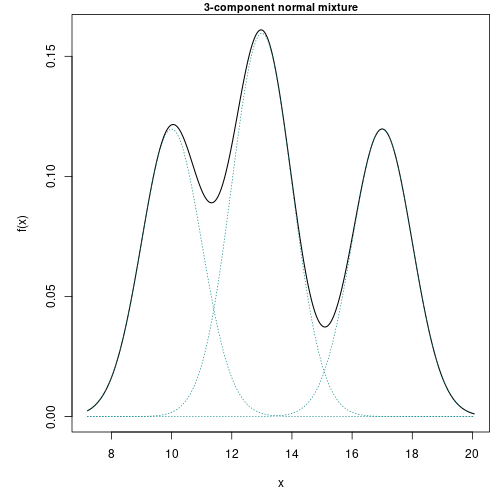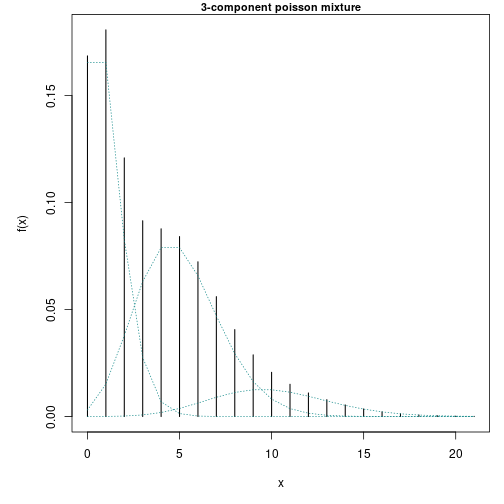If required, random samples can be generated from these mixtures.

# generate random samples:
normLocRMix <- rMix(1000, obj = normLocMix)
poisRMix <- rMix(1000, obj = poisMix)


The histograms of the generated random samples can be plotted.

# plot the histograms of the random samples:
par(mar = c(5, 5, 1, 1))
plot(normLocRMix, main = "Three component normal mixture", cex.main = 0.9)
plot(poisRMix, main = "Three component poisson mixture", cex.main = 0.9)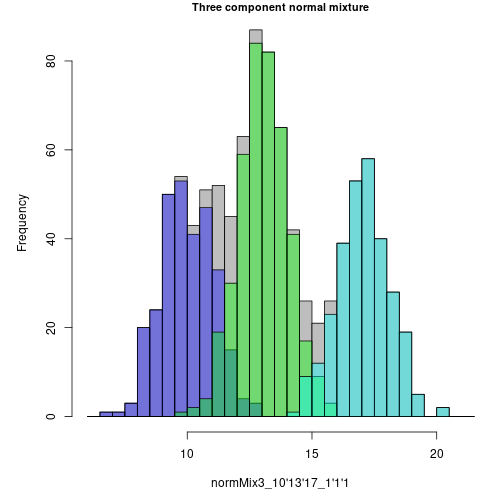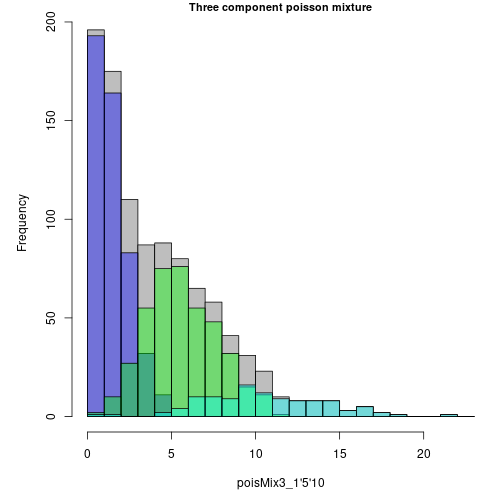The third object class shown in Table 1, called datMix, represents the data vector $$\textbf{X}$$ based on which the mixture complexity is supposed to be estimated. These objects are most central to the package, as every procedure estimating the order of a mixture takes a datMix object as input. Apart from $$\mathbf{X}$$, it contains other “static” information needed for the estimation procedure (in contrast to “tuning parameters”, which can be changed with every function call. An example of such a tuning parameter is the number of bootstrap replicates for a function employing a bootstrap procedure). A brief overview of which “static” attributes need to be supplied for each complexity estimation routine is given in Table 2.

#### Table 2: Inputs needed for different functions contained in mixComp

R function dist discrete theta.bound.list MLE.function Hankel.method Hankel.function
nonparamHankel x x x
nonparamHankel(.scaled) x x x o + i (optional) x x
L2(.boot).disc x x x i (optional)
hellinger(.boot).disc x x x i (optional)
hellinger(.boot).cont x x x i (optional)
mix.lrt x x x o + i (optional)

In the table, “o” and “i” respectively stand for input used during optimization and initialization. As a simple example of a given dataset to which mixture models have been applied extensively, take the Old Faithful dataset [@R; @faithful1; @faithful2]. In the context of mixture model estimation, the variable waiting, which gives the time in minutes between eruptions of the Old Faithful geyser in the Yellowstone National Park, is often considered to be the variable of interest. To estimate the number of components of the mixture distribution that provides a suitable approximation to the waiting data via mixComp, the raw data vector of observations has to be converted to a datMix object first. For the sake of exposition we specify all arguments of the datMix function, starting with the vector of observations $$\textbf{X}$$ and the string dist, specifying $$\{g(x;\theta):\theta \in \Theta \}$$ and the boolean discrete. As has often been done in the relevant literature, we assume that the data comes from a normal mixture.

faithful.obs <- faithful$waiting norm.dist <- "norm" norm.discrete <- FALSE  Second, a named list of length $$d$$ containing the bounds of $$\theta \in \Theta \subseteq \mathbf{R}^d$$ has to be created. In this example, $$\theta = \{\mu, \sigma\} \in \Theta = \mathbf{R} \times (0, \infty) \subseteq \mathbf{R}^2$$. # define the range for parameter values: norm.bound.list <- list("mean" = c(-Inf, Inf), "sd" = c(0, Inf))  Next, the argument MLE.function contains a single function if $$d = 1$$ or a list of functions of length $$d$$ otherwise, specifying the $$d$$ functions needed to estimate the MLE's of $$\theta$$ based on $$\textbf{X}$$ if $$p$$ were equal to 1 (i.e. the MLE's of the component distribution). If this argument is supplied and the datMix object is handed over to a complexity estimation procedure relying on optimizing over a likelihood function, the MLE.function attribute will be used for the single component case. In case the objective function is either not a likelihood or corresponds to a mixture with more than $$1$$ component, numerical optimization will be used based on Rsolnp's function solnp [@solnp, @Rsolnp]. The initial values (for the parameters of a $$j$$-component mixture, say) supplied to the solver are then calculated as follows: the data $$\textbf{X}$$ is clustered into $$j$$ groups by the function clara (of the cluster package by [@cluster] and the data corresponding to each group is given to MLE.function. The size of the groups is taken as initial component weights and the MLE's are taken as initial component parameter estimates. Specifying MLE.function is optional and if it is not, for example because the MLE solution does not exists in closed form, numerical optimization is used to find the relevant MLE's. Presuming a normal mixture, one specifies $$2$$ functions, namely the MLE of the mean $$\hat{\mu}_{MLE} = \frac{1}{n}\sum_{i = 1}^n X_i$$ and the MLE of the standard deviation $$\hat{\sigma}_{MLE} = \sqrt{\frac{1}{n}\sum_{i = 1}^n (X_i - \hat{\mu}_{MLE})^2}$$. # define the MLE functions for the mean and sd: MLE.norm.mean <- function(dat) mean(dat) MLE.norm.sd <- function(dat){ sqrt((length(dat) - 1) / length(dat)) * sd(dat) } MLE.norm.list <- list("MLE.norm.mean" = MLE.norm.mean, "MLE.norm.sd" = MLE.norm.sd)  The last two arguments, Hankel.method and Hankel.function, need to be supplied if the mixture complexity is to be estimated based on the Hankel matrix of the moments of the mixing distribution. The reader is referred to the Section 3 for further information on how these arguments are to be specified (in this case, the simplifying assumption of unit variance is made. This would be a poor choice for the waiting data, so $$p$$ should not be estimated with one of the methods using these arguments, namely nonparamHankel, paramHankel and paramHankel.scaled, see Table 2). method <- "translation" # define the function for computing the moments: mom.std.norm <- function(j){ ifelse(j %% 2 == 0, prod(seq(1, j - 1, by = 2)), 0) }  Finally, all previously generated objects are combined to a datMix object. # construct a datMix object that summarizes all the necessary information: faithful.dM <- datMix(faithful.obs, dist = norm.dist, discrete = norm.discrete, theta.bound.list = norm.bound.list, MLE.function = MLE.norm.list, Hankel.method = method, Hankel.function = mom.std.norm)  In the preceding example, the data vector $$\textbf{X}$$ was taken from an already existing dataset. As seen before, the rMix function can be used to generate a $$n$$-sized sample from a specific mixture. If this synthesized data is to be used in simulations (i.e. passed to one of the functions estimating the mixture complexity) an rMix object can be converted to a datMix object via the RtoDat function. Apart from dist and discrete, all datMix arguments have to be supplied to RtoDat likewise. We will introduce the relevant examples in Section 3. Unlike the above mentioned objects whose creation precedes any type of mixture complexity estimation, objects of the bottom two classes (see Table 1) contain the results of the estimation procedures. Generally, the functions estimating the number of components differ in the types of families of component densities $$\{g(x;\theta):\theta \in \Theta \}$$ for which they allow and in whether they provide estimates of the weights $$\hat{w}_i$$ and the component parameters $$\hat{\theta}_i, i \in 1, \dots, \hat{p}$$, the latter determining the object class of the estimation result. These differences are shown in Table 3. The function nonparamHankel returns an object of class hankDet, which is a vector of determinants (scaled and/or penalized), each entry belonging to a certain complexity estimate. The link between these determinants and $$p$$ will be discussed in the Section 3. paramEst objects arise when using any other function estimating the mixture complexity, all of which additionally return estimates of the component weights and parameters. For both object classes, print and plot methods are available to summarize and visualize the estimation results. #### Table 3: Distribution restrictions and output types of different functions contained in mixComp R function Restrictions on the family of the component density Estimation of $$\mathbf{w}$$ and $$\mathbf{\theta}$$ nonparamHankel Compatible with explicit, translation or scale nonparamHankel(.scaled) Compatible with explicit, translation or scale x L2(.boot).disc Discrete distributions x hellinger(.boot).disc Discrete distributions x hellinger(.boot).cont Continuous distributions x mix.lrt x ## Section 3. Functions using Hankel matrices In 1997, [@hankel] showed that the order of a mixture $$p$$ is characterized by the smallest integer $$j$$ such that the determinant of the $$(j+1) \times (j+1)$$ Hankel matrix of the first $$2j$$ moments of the mixing distribution equals zero. Moreover, it can be shown that this determinant is zero for all $$j \geq p$$. Formally, for any vector $$\mathbf{c} = (c_0, \dots, c_{2k}) \in \mathbb{R}^{2k+1}$$ with $$c_0$$ equal to 1, the Hankel matrix of $$\mathbf{c}$$ is defined as the $$(k+1)\times(k+1)$$ matrix given by $H(\mathbf{c})_{i,j} = c_{i+j-2}, \quad \quad 1 \leq i,j \leq k+1.$ Now, let $$\mathbf{c}^{2j+1} \in \mathbb{R}^{2j+1}$$ be the vector containing the first $$2j+1$$ (raw) moments of the mixing distribution. For finite mixture models, this amounts to $c^{2j+1}_k = \sum_{i=1}^p w_i \theta^k_i, \quad \text{ for } k \in \{0,\dots, 2j\} \text{ and } \theta_i \in \mathbb{R}, i \in \{1,\dots,p\}.$ Then, for all $$j \geq 1$$, $$H(\textbf{c}^{2j+1})$$ is non-negative and $p = \min\{j \geq 1 : \det H(\textbf{c}^{2j+1}) = 0\}.$ Making use of this fact, the first approach to estimating the order of a mixture that is implemented in mixComp relies on initially finding a consistent estimator of $$\textbf{c}^{2j+1}$$ based on $$\textbf{X}$$, say $$\hat{\textbf{c}}^{2j+1}$$, to then iteratively calculate the applicable Hankel matrix while increasing the assumed order $$j$$ until a sufficiently small value of $$\det H(\hat{\textbf{c}}^{2j+1})$$ is attained. However, since $$\det H(\hat{\textbf{c}}^{2j+1})$$ should be close to 0 for all $$j \geq p$$, this would lead to choosing $$\hat{p}$$ rather larger than the true value and it seems natural to introduce a penalty term. Therefore [@hankel] define the empirical penalized objective function as $J_n(j) := \lvert \det H(\hat{\mathbf{c}}^{2j+1}) \rvert + A(j)l(n),$ with $$l(n)$$ being a positive function converging to $$0$$ as $$n\to\infty$$ and $$A(j)$$ being positive and strictly increasing. $\hat{p} := \arg\min_{j \in \mathbb{N}} J_n(j)$ is then a consistent estimator of $$p$$. As an extension to simply adding a penalty term to the determinant, a scaling approach was considered by [@lilian]. Let $$\hat{d}_j = \det H(\hat{\mathbf{c}}^{2j+1})$$, $$d_j = \det H(\mathbf{c}^{2j+1})$$ and $$j_m \geq p, j_m \in \mathbb{N}$$. Since the estimated moments $$\hat{\mathbf{c}}^{2j+1}$$ are asymptotically normal, one can apply the delta method giving $\sqrt{n} \cdot \big( \hat{d}_1-d_1, \dots, \hat{d}_{p-1}-d_{p-1}, \hat{d}_p-0, \dots, \hat{d}_{j_m}-0 \big)^\top \quad \overset{\mathcal{D}}{\longrightarrow} \quad \mathcal{N}(0_{j_m \times 1}, \Sigma_ {j_m \times j_m}).$ Instead of inspecting the vector $$(\hat{d}_1, \dots, \hat{d}_{j_m})^T$$, one could therefore also base the complexity analysis on a vector of scaled determinants, employing a nonparametric bootstrap procedure on $$\mathbf{X}$$. To this end, let $$\tilde{\Sigma} \in \mathbb{R}^{j_m \times j_m}$$ denote the covariance matrix of the determinants $$\hat{d}^{*b}_{j}$$ calculated on the $$b^{\text{th}}$$ bootstrap sample for $$b=1, \dots, B$$ and $$j = 1, \dots j_m$$. Note that $\tilde{\Sigma} \to \frac{\Sigma}{n} \quad \text{ as }B \to \infty, n \to \infty$ and write $$\tilde{\Sigma}^{-½} = \sqrt{n} \cdot \hat{\Sigma}^{-½}$$. Define the rescaled vector $\big( y_1, \dots, y_p, \dots, y_{j_m} \big)^\top := \sqrt{n} \cdot {\hat{\Sigma}}^{-½} \big( \hat{d}_1, \dots, \hat{d}_p, \dots, \hat{d}_{j_m} \big)^\top.$ Note that in the case of the scaled version, the criterion to be minimized becomes ${J_n(j)}_{scaled} := \vert y_j \vert + A(j)l(n) \cdot \sqrt{n}.$ That is, the chosen penalty function should be multiplied by $$\sqrt{n}$$. This approach was proposed to address the issue of determinants already being very small from the beginning (even for $$j = 1$$), which, in the simulations by [@lilian], made it hard to discern the “best” complexity estimate, a problem that was not reduced much by solely adding a penalty term. With this general framework in place, the computation now merely hinges on calculating $$\hat{\textbf{c}}^{2j+1}$$. The mixComp package offers three methods to do so. The method to use depends on the family of component densities $$\{g(x;\theta):\theta \in \Theta \}$$ and is linked to some function $$f_j(\mathbf{X})$$ needed to estimate $$\hat{\mathbf{c}}^{2j+1}$$. The calculation method and the relevant function are specified when creating the datMix object as arguments Hankel.method and Hankel.function. #### 1. Hankel.method = "explicit" This method can be applied when a closed form expression for estimates of the moments of the mixing distribution exists. Hankel.function then contains the function explicitly estimating $$\mathbf{c}^{2j+1}$$. As an example, consider a mixture of geometric distributions, where it can be shown that $c^{2j+1}_j = 1 - \sum_{l = 0}^{j-1} f(l) = 1 - F(j-1),$ with $$F$$ the true cumulative distribution function. This expression is called $$c^{2j+1}_j$$ only for notational consisteny; the RHS is simply a closed-form expression for the $$j^{th}$$ moment of the mixing distribution of a geometric mixture. Hence one may take $\hat{c}^{2j+1}_j = 1 - \hat{F}(j-1)$ as an estimator, with $$\hat{F}$$ being the empirical distribution function. # define the function for computing the moments: explicit.geom <- function(dat, j){ 1 - ecdf(dat)(j - 1) }  As a second example, consider what [@hankel, p. 283, equation (3)] called the “natural” estimator, i.e. using $\hat{c}^{2j+1}_j = f_j\left(\frac{1}{n} \sum_{i=1}^n \psi_j(X_i)\right)$ when $c^{2j+1}_j = f_j(\mathbb{E}[\psi_j(X_i)]).$ Note that the estimators of this form may also be supplied as Hankel.method = "explicit" with Hankel.function. For example, the “natural” estimator is applicable in the case of Poisson mixtures. If $$Y \sim Pois(\lambda)$$, it is a well known fact that $\lambda^j = \mathbb{E}[Y(Y-1)\dots(Y-j+1)],$ which then suggests using $\hat{c}^{2j+1}_j = \frac{1}{n} \sum_{i=1}^n X_i(X_i-1)\dots(X_i-j+1)$ as an estimator. # define the function for computing the moments: explicit.pois <- function(dat, j){ mat <- matrix(dat, nrow = length(dat), ncol = j) - matrix(0:(j-1), nrow = length(dat), ncol = j, byrow = TRUE) return(mean(apply(mat, 1, prod))) }  #### 2. Hankel.method = "translation" Example 3.1. in [@hankel, p.284] describes how $$\textbf{c}^{2j+1}$$ can be estimated if the family of component distributions $$(G_\theta)$$ is given by $$\text{d}G_\theta(x) = \text{d}G(x-\theta)$$, where $$G$$ is a known probability distribution whose moments can be given explicitly. In this case, a triangular linear system can be solved for the estimated moments of the mixing distribution $$\hat{\textbf{c}}^{2j+1}$$ using the empirical moments of the mixture distribution and the known moments of $$G$$. The former can be estimated from the data vector $$\textbf{X}$$ whereas the latter has to be supplied by the user. Thus, Hankel.function contains a function of $$j$$ returning the $$j^{th}$$ (raw) moment of $$G$$. As an example, consider a mixture of normal distributions with unknown mean and unit variance. Then $$G$$ is the standard normal distribution, and its $$j^{th}$$ moment $$m_j$$ is defined as $m_j= \begin{cases} 0 & \text{if } j \text{ uneven}\ (j-1)!! & \text{if } j \text{ even} .\end{cases}$ # define the function for computing the moments: mom.std.norm <- function(j){ ifelse(j %% 2 == 0, prod(seq(1, j - 1, by = 2)), 0) }  #### 3. Hankel.method = "scale" Similarly, example 3.2. in [@hankel, p.285] describes how $$\textbf{c}^{2j+1}$$ can be estimated if the family of component distributions $$(G_\theta)$$ is given by $$\text{d}G_\theta(x) = \text{d}G(\frac{x}{\theta})$$, where $$G$$ is a known probability distribution whose moments can be given explicitly. Likewise, a triangular linear system can be solved for $$\hat{\textbf{c}}^{2j+1}$$, using the empirical moments of the mixture distribution and the known moments of $$G$$. Hankel.function contains a function of $$j$$ returning the $$j^{th}$$ moment of $$G$$. Note that squares have to be taken everywhere if for some integer $$j$$, $$m_j = 0$$ (compare with [@hankel, p.285]). As an example, consider a mixture of normal distributions with zero mean and unknown variance. Then $$G$$ is again the standard normal distribution, and its $$j^{th}$$ moment is defined as above. Coming back to the overall goal of complexity estimation, the function nonparamHankel returns all estimated determinant values corresponding to complexities up to j.max, so that the user can pick the lowest $$j$$ generating a sufficiently small determinant. The function allows the inclusion of a penalty term as a function of the sample size n and the currently assumed complexity j which will be added to the determinant value (by supplying pen.function), and/or scaling of the determinants (by setting scaled = TRUE). For scaling, a nonparametric bootstrap is used to calculate the covariance of the estimated determinants, with B being the size of the bootstrap sample. The inverse of the square root (i.e. the matrix $$S$$ such that $$A = SS$$, where $$A$$ is the (square) covariance matrix. The procedure uses expm's sqrtm [@expm]) of this covariance matrix is then multiplied with the estimated determinant vector to get the scaled determinant vector. We will initially apply this method to the two already generated datasets of $$3$$-component Poisson and normal mixtures using the penalty $$A(j)l()n = \frac{j \log(n)}{\sqrt{n}}$$ and scaling the determinants as described above. First, for converting the previously simulated samples from $$3$$-component Poisson and normal mixtures yielding the objects of class rMix to objects of class datMix one should apply the RtoDat function as follows: MLE.pois <- function(dat) mean(dat) # create datMix objects: pois.dM <- RtoDat(poisRMix, theta.bound.list = list(lambda = c(0, Inf)), MLE.function = MLE.pois, Hankel.method = "explicit", Hankel.function = explicit.pois) normLoc.dM <- RtoDat(normLocRMix, theta.bound.list = norm.bound.list, MLE.function = MLE.norm.list, Hankel.method = "translation", Hankel.function = mom.std.norm)  In the case of the scaled version of the method, the penalty should be multiplied by $$\sqrt{n}$$ as mentioned earlier. # define the penalty function: pen <- function(j, n){ j * log(n) } # apply the nonparamHankel function to the datMix objects: set.seed(1) poisdets_sca_pen <- nonparamHankel(pois.dM, j.max = 5, scaled = TRUE, B = 1000, pen.function = pen) normdets_sca_pen <- nonparamHankel(normLoc.dM, j.max = 5, scaled = TRUE, B = 1000, pen.function = pen)  We can print and plot the results as suggested below. # print the results (for the Poisson mixture) print(poisdets_sca_pen) #< #< Estimation of the scaled and penalized determinants for a 'pois' mixture model: #< Number of components Determinant #< 1 20.23365 #< 2 13.82135 #< 3 20.90053 #< 4 27.70596 #< 5 34.54453 # plot results for both mixtures: par(mar = c(5, 5, 1, 1)) plot(poisdets_sca_pen, main = "3-component Poisson mixture", cex.main = 0.9) plot(normdets_sca_pen, main = "3-component Normal mixture", cex.main = 0.9)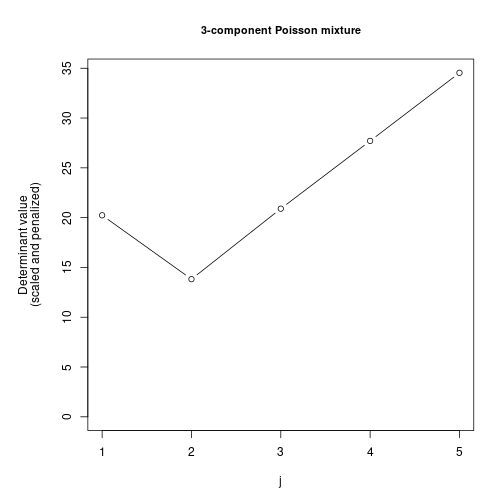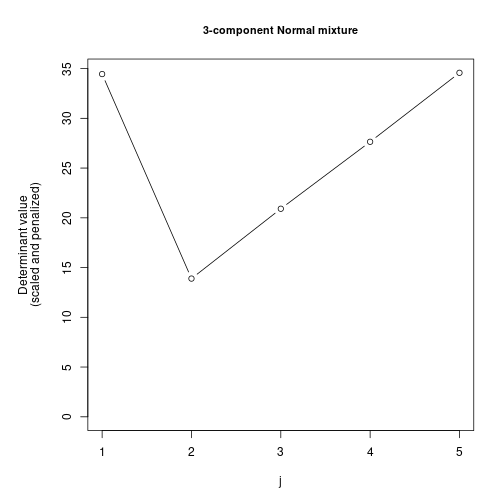The resulting plots indicate that while theoretically sound, the scaled version of the Hankel method can struggle to correctly identify the number of components in practice. As the preceding example shows, it can be quite difficult to determine the order estimate from the vector of estimated determinants alone. Thus, the package includes another option of estimating $$p$$ based on Hankel matrices, however, using a more “parametric” approach which goes hand in hand with estimating $$\mathbf{w}$$ and $$\mathbf{\theta}$$. The paramHankel procedure initially assumes the mixture to only contain a single component, setting $$j = 1$$, and then sequentially tests $$p = j$$ versus $$p = j+1$$ for $$j = 1,2, \dots$$, until the algorithm terminates. To do so, it determines the MLE for a $$j$$-component mixture $$(\hat{\mathbf{w}}^j, \hat{\mathbf{\theta}}^j) = (\hat{w}_1, \dots, \hat{w}_j, \hat{\theta}_1, \dots, \hat{\theta}_j) \in W_j \times \Theta_j$$, generates B parametric bootstrap samples of size $$n$$ from the distribution corresponding to $$(\hat{\mathbf{w}}^j, \hat{\mathbf{\theta}}^j)$$ and calculates B determinants of the corresponding $$(j+1) \times (j+1)$$ Hankel matrices. The null hypothesis $$H_0: p = j$$ is rejected and $$j$$ increased by $$1$$ if the determinant value based on the original data vector $$\textbf{X}$$ lies outside of the interval $$[ql, qu]$$, a range specified by the ql and qu empirical quantiles of the bootstrapped determinants. Otherwise, $$j$$ is returned as the order estimate $$\hat{p}$$. paramHankel.scaled functions similarly to paramHankel with the exception that the bootstrapped determinants are scaled by the empirical standard deviation of the bootstrap sample. To scale the original determinant, B nonparametric bootstrap samples of size $$n$$ are generated from the data, the corresponding determinants are calculated and their empirical standard deviation is used. Applying paramHankel.scaled to the same Poisson and Normal mixtures results in the correct identification of the mixture complexity in both cases as can be seen in the plot: # apply papamHankel.scaled to datMix objects: set.seed(1) pois_sca_pen <- paramHankel.scaled(pois.dM) #< #< Parameter estimation for a 1 component 'pois' mixture model: #< Function value: 3008.6529 #< w lambda #< Component 1: 1 3.661 #< Optimization via user entered MLE-function. #< ---------------------------------------------------------------------- #< #< Parameter estimation for a 2 component 'pois' mixture model: #< Function value: 2439.6581 #< w lambda #< Component 1: 0.53139 1.1141 #< Component 2: 0.46861 6.5491 #< Converged in 3 iterations. #< ---------------------------------------------------------------------- #< #< Parameter estimation for a 3 component 'pois' mixture model: #< Function value: 2401.5429 #< w lambda #< Component 1: 0.455849 0.8742 #< Component 2: 0.464642 5.1340 #< Component 3: 0.079509 11.0305 #< Converged in 3 iterations. #< ---------------------------------------------------------------------- #< #< The estimated order is 3. norm_sca_pen <- paramHankel.scaled(normLoc.dM) #< #< Parameter estimation for a 1 component 'norm' mixture model: #< Function value: 2477.8089 #< w mean sd #< Component 1: 1.00 13.31 2.8831 #< Optimization via user entered MLE-function. #< ---------------------------------------------------------------------- #< #< Parameter estimation for a 2 component 'norm' mixture model: #< Function value: 2388.9577 #< w mean sd #< Component 1: 0.75885 12.04949 2.0269 #< Component 2: 0.24115 17.27588 0.9020 #< Converged in 3 iterations. #< ---------------------------------------------------------------------- #< #< Parameter estimation for a 3 component 'norm' mixture model: #< Function value: 2357.6367 #< w mean sd #< Component 1: 0.44170 13.01595 1.0613 #< Component 2: 0.28142 17.09978 1.0013 #< Component 3: 0.27688 9.92665 1.0113 #< Converged in 3 iterations. #< ---------------------------------------------------------------------- #< #< The estimated order is 3. # plot the results for both mixtures: par(mar=c(5, 5, 1, 1)) plot(pois_sca_pen,) plot(norm_sca_pen)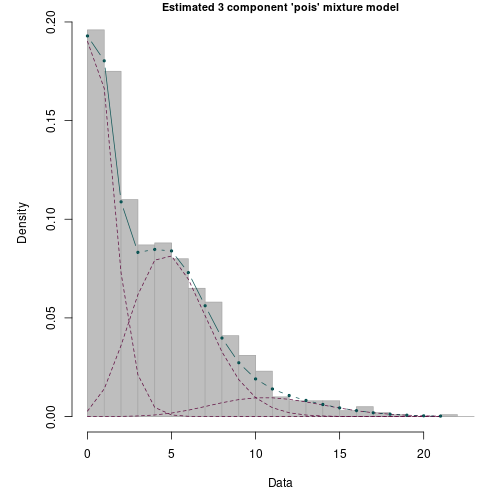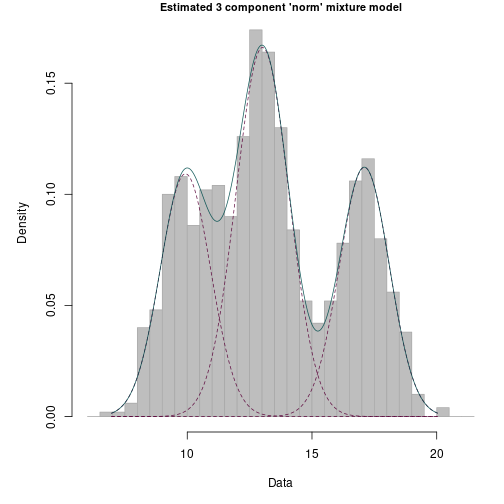As another example, consider data generated from a three component geometric mixture, with $$\mathbf{w} = (0.1, 0.6, 0.3)$$ and $$\mathbf{\theta} = (0.8, 0.2, 0.4)$$. set.seed(1) # define the geometric mixture: geomMix <- Mix("geom", discrete=TRUE, w = c(0.1, 0.6, 0.3), prob = c(0.8, 0.2, 0.4)) # generate a random sample from the mixture: geomRMix <- rMix(1000, obj = geomMix) # construct the corresponding datMis object: MLE.geom <- function(dat){ 1/(mean(dat)+1) } geom.dM <- RtoDat(geomRMix, Hankel.method = "explicit", Hankel.function = explicit.geom, theta.bound.list = list(prob = c(0, 1)), MLE.function = MLE.geom) # etimate the number of components using paramHankel function: (res <- paramHankel(geom.dM, j.max = 5, B = 1000, ql = 0.025, qu = 0.975)) #< #< Parameter estimation for a 1 component 'geom' mixture model: #< Function value: 2269.5588 #< w prob #< Component 1: 1 0.2456 #< Optimization via user entered MLE-function. #< ---------------------------------------------------------------------- #< #< Parameter estimation for a 2 component 'geom' mixture model: #< Function value: 2240.6233 #< w prob #< Component 1: 0.22916 0.7643 #< Component 2: 0.77084 0.2044 #< Converged in 3 iterations. #< ---------------------------------------------------------------------- #< #< Parameter estimation for a 3 component 'geom' mixture model: #< Function value: 2239.5088 #< w prob #< Component 1: 0.11538 1.0000 #< Component 2: 0.57009 0.1844 #< Component 3: 0.31454 0.3640 #< Converged in 3 iterations. #< ---------------------------------------------------------------------- #< #< The estimated order is 3. #< #< Parameter estimation for a 3 component 'geom' mixture model: #< Function value: 2239.5088 #< w prob #< Component 1: 0.11538 1.0000 #< Component 2: 0.57009 0.1844 #< Component 3: 0.31454 0.3640 #< Converged in 3 iterations. #< #< The estimated order is 3.  Again, paramHankel correctly identifies the data as having been generated by a $$3$$-component mixture. Consider now, as a real-world example, the Children dataset whose content was taken from the Annual Report of the pension fund S.P.P. of 1952. The dataset initially appeared in work of [@thisted] and was subsequently analysed by many authors. It entails data on 4075 widows who recieved pension from the fund, with their number of children being our variable of interest. For example, there are 3062 widows without children, 587 widows with one child, etc. Many authors have noted that this data is not consistent with being a random sample from a Poisson distribution since the number of zeros found in the data is too large. Thisted approached this by fitting a mixture of two populations, one which is always zero and one which follows a Poisson distribution. mixComp includes this data stored as a dataframe. Here, we want to investigate how the Hankel matrix methods compare when fitting the data to a mixture of Poissons. The estimation process starts with the construction of the datMix object. # convert the data to vetor: children.obs <- unlist(children) # define the MLE function: MLE.pois <- function(dat) mean(dat) # construct a datMix object: children.dM <- datMix(children.obs, dist = "pois", discrete = TRUE, Hankel.method = "explicit", Hankel.function = explicit.pois, theta.bound.list = list(lambda = c(0, Inf)), MLE.function = MLE.pois)  First, we check the nonparametric method. We define the penalty $$A(j)l(n)$$ as $$j\log(n)$$ (by multiplying $$\frac{j\log(n)}{\sqrt{n}}$$ by $$\sqrt{n}$$). The result suggests that the data comes from a 2-component mixture. # define the penalty: pen <- function(j, n) j * log(n) # estimate the number of components: set.seed(0) (det_sca_pen <- nonparamHankel(children.dM, j.max = 5, scaled = TRUE, B = 1000, pen.function = pen)) #< #< Estimation of the scaled and penalized determinants for a 'pois' mixture model: #< Number of components Determinant #< 1 21.61041 #< 2 17.15443 #< 3 25.60157 #< 4 33.67663 #< 5 41.79636 #plot the results: plot(det_sca_pen, main = "Non-parametric Hankel method for Children dataset", cex.main = 0.9)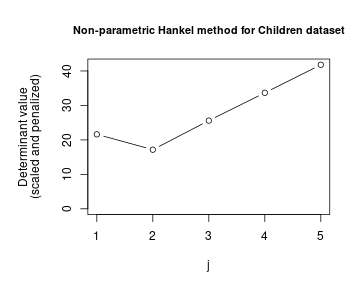Next, we check the fit of the parametric version. The printed result of paramHankel.scaled shows that this method also suggests 2 to be the number of components, with the first component corresponding to a Poisson distribution with $$\lambda = 0.0306$$. Note that the limit case $$\lambda = 0$$ results in a point mass at $$0$$, and that this fit therefore nicely lines up with the idea of a component accounting for only the zero observations. The plot shows that this method yields a sensible fit overall. set.seed(0) param_sca <- paramHankel.scaled(children.dM, j.max = 5, B = 1000, ql = 0.025, qu = 0.975) #< #< Parameter estimation for a 1 component 'pois' mixture model: #< Function value: 3640.3094 #< w lambda #< Component 1: 1 0.3995 #< Optimization via user entered MLE-function. #< ---------------------------------------------------------------------- #< #< Parameter estimation for a 2 component 'pois' mixture model: #< Function value: 3350.9289 #< w lambda #< Component 1: 0.65959 0.0306 #< Component 2: 0.34041 1.1143 #< Converged in 3 iterations. #< ---------------------------------------------------------------------- #< #< The estimated order is 2. plot(param_sca, breaks = 8, ylim = c(0, 0.8))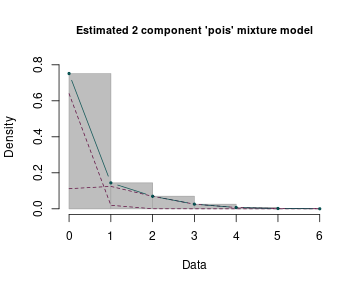## Section 4. Functions using distances Unlike the theory on Hankel matrices introduced in Section 3, many theoretical considerations rely on estimates of the weights $$\mathbf{w}$$ and the component parameters $$\mathbf{\theta}$$. As mentioned in the introduction, it is assumed that the family of component densities $$\{g(x;\theta): \theta \in \Theta \}$$ is known. To embed the subsequent algorithms in a more theoretical framework, consider the parametric family of mixture densities $\mathcal{F}_j = \{ f_{j, \mathbf{w},\mathbf{\theta}} : (\mathbf{w}, \mathbf{\theta}) \in W_j \times \Theta_j \}.$ With $$\{g(x;\theta): \theta \in \Theta \}$$ set in advance, elements of $$\mathcal{F}_j$$ can be written as $f_{j,\mathbf{w},\mathbf{\theta}}(x) = \sum_{i = 1}^j w_i g(x; \theta_i).$ Note that the support of $$f$$ will depend on the support of $$g$$ and $$\mathcal{F}_j \subseteq \mathcal{F}_{j+1}$$ (This can be seen by setting $$w_{j+1} = 0$$) for all $$j$$. Now take a specific mixture $$f_0 = f_{p_0, \mathbf{w}_0,\mathbf{\theta}_0}$$, where $$(\mathbf{w}_0,\mathbf{\theta}_0) \in W_{p_0} \times \Theta_{p_0}$$. Clearly, the mixture's complexity is defined as $p_0 = \min\{j:f_0 \in \mathcal{F}_j\}.$ The above suggests an estimation procedure based on initially finding the “best” possible estimate (in a sense to be determined) $$(\hat{\mathbf{w}}^j, \hat{\mathbf{\theta}}^j) \in W_j \times \Theta_j$$ for a given value of $$j$$, in order to compare the thereby specified pdf/pmf $\hat{f}_j(x) = f_{j, \hat{\mathbf{w}}^j, \hat{\mathbf{\theta}}^j}(x),$ with a non-parametric density/probability mass estimate $$\tilde{f}_n(x)$$. As the classes $$\mathcal{F}_j$$ and $$\mathcal{F}_{j+1}$$ are nested, the distance $$D$$ (to be defined below) between $$\hat{f}_j$$ and $$\tilde{f}_n$$ will not increase with $$j$$. Thus, it makes sense to add some penalty term (increasing in $$j$$) to $$D(\hat{f}_j, \tilde{f}_n)$$ and find the first value of $$j$$ where the penalized distance for $$j$$ is smaller than that for $$j+1$$. Rearranging the terms gives rise to an algorithm starting at $$j = 1$$, involving some threshold $$t(j,n)$$ depending on the penalty, where, if $$j$$ is the first integer satisfying $D(\hat{f}_j, \tilde{f}_n) - D(\hat{f}_{j+1}, \tilde{f}_n) \leq t(j,n),$ then $$j$$ is taken as the estimate $$\hat{p}$$. If the inequality is not fulfilled, $$j$$ is increased by $$1$$ and the procedure is repeated. Consistency of estimators defined this way has been shown in a number of cases, amongst them those used by the mixComp algorithms, and the reader is referred to [@l2; @hell; @hellcont] for the proofs relevant to the results implemented in the package. The preceding notation was held as broad as possible, since different distance measures $$D$$ and non-parametric estimators $$\tilde{f}_n$$ can be used. Those relevant to the package are mostly well-known, still, definitions can be found in the Appendix. Three procedures are implemented in mixComp based on the foregoing methodology: L2.disc, hellinger.disc and hellinger.cont. #### 1. L2.disc L2.disc employs the squared $$L_2$$ distance as the distance measure $$D$$ and is only to be used for discrete mixtures since the nonparametric estimate $$\tilde{f}_n$$ is defined as the empirical probability mass function. In this setting, the “best” estimate $(\hat{\mathbf{w}}^j, \hat{\mathbf{\theta}}^j) \in W_j \times \Theta_j$ for a given $$j$$ corresponds to $(\hat{\mathbf{w}}^j, \hat{\mathbf{\theta}}^j) = \arg\min_{(\mathbf{w}, \mathbf{\theta})} L_2^2(f_{j, \mathbf{w}, \mathbf{\theta}}, \hat{f_n}) = \arg\min_{(\mathbf{w}, \mathbf{\theta})} \left\{ \sum_{x=0}^{\infty} f_{j, \mathbf{w}, \mathbf{\theta}}^2(x) - \frac{2}{n} \sum_{i=1}^{n}f_{j, \mathbf{w}, \mathbf{\theta}}(X_i)\right\}.$ As the squared $$L_2$$ distance might involve an infinite sum (for distributions with infinite support), the user has the option to determine the cut-off value using the n.inf argument, which is set to 1000 by default. The parameters $$(\hat{\mathbf{w}}^{j+1}, \hat{\mathbf{\theta}}^{j+1})$$ are obtained analogously. Once both parameter sets have been determined, the difference in their respective squared $$L_2$$ distances to $$\tilde{f}_n$$ is compared to a threshold (equaling $$t(j,n)$$ defined above. The threshold function can be entered directly or one of the predefined thresholds, called LIC or SBC and given respectively by $\frac{0.6}{n} \ln\left(\frac{j+1}{j}\right) \quad\quad \text{ and} \quad\quad \frac{0.6 \ln(n)}{n} \ln\left(\frac{j+1}{j}\right)$ can be used. Note that, if a customized function is to be used, its arguments have to be named j and n. If the difference in squared distances is smaller than the selected threshold, the algorithm terminates and the true order is estimated as $$j$$, otherwise $$j$$ is increased by $$1$$ and the procedure starts over. The reader is invited to consult [@l2] for further details. #### 2. hellinger.disc This second function presents an alternative estimation procedure for discrete mixtures, working much the same as L2.disc, however, using a different measure of distance and different thresholds. As the name suggests, it is based on the square of the Hellinger distance, causing the “best” estimate $$(\hat{\mathbf{w}}^j, \hat{\mathbf{\theta}}^j) \in W_j \times \Theta_j$$ for a given $$j$$ to equal $(\hat{\mathbf{w}}^j, \hat{\mathbf{\theta}}^j) = \arg\min_{(\mathbf{w}, \mathbf{\theta})} H^2(f_{j, \mathbf{w}, \mathbf{\theta}}, \hat{f_n}) = \arg\max_{(\mathbf{w}, \mathbf{\theta})} \sum_{x=0}^{X_{(n)}} \sqrt{f_{j, \mathbf{w}, \mathbf{\theta}}(x) \tilde{f}_n(x)},$ with $$X_{(n)} = \max_{i = 1}^n (X_i)$$. The relevant theory can be found in [@hell]. In accordance with their work, the two predefined thresholds are given by $\text{AIC} = \frac{d+1}{n} \quad \quad \text{and} \quad \quad \text{SBC} = \frac{(d+1)\ln(n)}{2n}$ (recall that $$d$$ is the number of component parameters, i.e. $$\Theta \subseteq \mathbb{R}^d$$). If a customized function is to be used, its arguments have to named j and n once more, so if the user wants to include the number of component parameters $$d$$, it has to be entered explicitly. #### 3. hellinger.cont Unlike the two preceding functions, this procedure is applicable to continuous mixture models and uses a kernel density estimator (KDE) as $$\tilde{f}_n$$. Its bandwidth can be chosen by the user, or the adaptive KDE found in [@adap, p. 1720, equation (2)] may be used by specifying bandwidth = "adaptive". The calculations are based on the continuous version of the squared Hellinger distance, where the “best” estimate $$(\hat{\mathbf{w}}^j, \hat{\mathbf{\theta}}^j) \in W_j \times \Theta_j$$ for a given $$j$$ corresponds to $(\hat{\mathbf{w}}^j, \hat{\mathbf{\theta}}^j) = \arg\min_{(\mathbf{w}, \mathbf{\theta})} H^2(f_{j, \mathbf{w}, \mathbf{\theta}}, \hat{f_n}) = \arg\max_{(\mathbf{w}, \mathbf{\theta})} \int \sqrt{f_{j, \mathbf{w}, \mathbf{\theta}}(x)\tilde{f}_n(x)}\ dx.$ Since the computational burden of optimizing over an integral to find the “best” weights and component parameters is immense, the algorithm approximates the objective function defined in the previous equation by sampling$n_s = $sample.n observations $$Y_i$$ from $$\tilde{f}_n(x)$$ and setting $(\hat{\mathbf{w}}^j, \hat{\mathbf{\theta}}^j) = \arg\max_{(\mathbf{w}, \mathbf{\theta})} \sum_{i = 1}^{n_s} \sqrt{\frac{f_{j, \mathbf{w}, \mathbf{\theta}}(Y_i)}{\tilde{f}_n(Y_i)}}.$ Consider again the artificially created samples from the 3-component normal mixture with $$\mathbf{w} = (0.3, 0.4, 0.3)$$, $$\mathbf{\mu} = (10, 13, 17)$$ and $$\mathbf{\sigma} = (1, 1, 1)$$ and the Poisson mixture with $$\mathbf{w} = (0.45, 0.45, 0.1)$$, $$\mathbf{\lambda} = (1, 5, 10)$$. This procedure hellinger.cont uses the same thresholds as hellinger.disc. set.seed(0) h_disc_pois <- hellinger.disc(pois.dM, threshold = "AIC") #< Warning: While being used in Woo & Sriram's original paper, asymptotically, the #< threshold 'AIC' does not go to 0 slower than the difference in squared Hellinger #< distances once the correct order p is reached and the estimator is therefore not #< consistent. #< #< Parameter estimation for a 1 component 'pois' mixture model: #< Function value: 0.2075 #< w lambda #< Component 1: 1 3.2407 #< Converged in 3 iterations. #< ---------------------------------------------------------------------- #< #< Parameter estimation for a 2 component 'pois' mixture model: #< Function value: 0.01573 #< w lambda #< Component 1: 0.51767 1.0411 #< Component 2: 0.48233 6.1802 #< Converged in 3 iterations. #< ---------------------------------------------------------------------- #< #< Parameter estimation for a 3 component 'pois' mixture model: #< Function value: 0.003166 #< w lambda #< Component 1: 0.452735 0.8622 #< Component 2: 0.089649 10.4139 #< Component 3: 0.457616 5.0285 #< Converged in 3 iterations. #< ---------------------------------------------------------------------- #< #< Parameter estimation for a 4 component 'pois' mixture model: #< Function value: 0.002919 #< w lambda #< Component 1: 0.400256 0.7607 #< Component 2: 0.385412 5.5813 #< Component 3: 0.144987 2.8377 #< Component 4: 0.069346 10.9255 #< Converged in 3 iterations. #< ---------------------------------------------------------------------- #< #< The estimated order is 3. h_cont_norm <- hellinger.cont(normLoc.dM, bandwidth = 0.5, sample.n = 5000, threshold = "AIC") #< Warning: While being used in Woo & Sriram's original paper, asymptotically, the #< threshold 'AIC' does not go to 0 slower than the difference in squared Hellinger #< distances once the correct order p is reached and the estimator is therefore not #< consistent. #< #< Parameter estimation for a 1 component 'norm' mixture model: #< Function value: 0.04901 #< w mean sd #< Component 1: 1.000 13.158 2.7974 #< Converged in 3 iterations. #< ---------------------------------------------------------------------- #< #< Parameter estimation for a 2 component 'norm' mixture model: #< Function value: 0.01098 #< w mean sd #< Component 1: 0.76055 11.97358 2.0732 #< Component 2: 0.23945 17.24876 0.9982 #< Converged in 3 iterations. #< ---------------------------------------------------------------------- #< #< Parameter estimation for a 3 component 'norm' mixture model: #< Function value: 0.001312 #< w mean sd #< Component 1: 0.47864 12.81608 1.2714 #< Component 2: 0.27343 17.08234 1.0797 #< Component 3: 0.24793 9.62003 1.0379 #< Converged in 3 iterations. #< ---------------------------------------------------------------------- #< #< Parameter estimation for a 4 component 'norm' mixture model: #< Function value: 0.001254 #< w mean sd #< Component 1: 0.441868 12.914312 1.1795 #< Component 2: 0.277960 17.055009 1.0948 #< Component 3: 0.196354 10.161401 1.0177 #< Component 4: 0.083818 8.911450 0.8360 #< Converged in 3 iterations. #< ---------------------------------------------------------------------- #< #< The estimated order is 3. par(mar = c(5, 5, 1, 1)) plot(h_disc_pois) plot(h_cont_norm)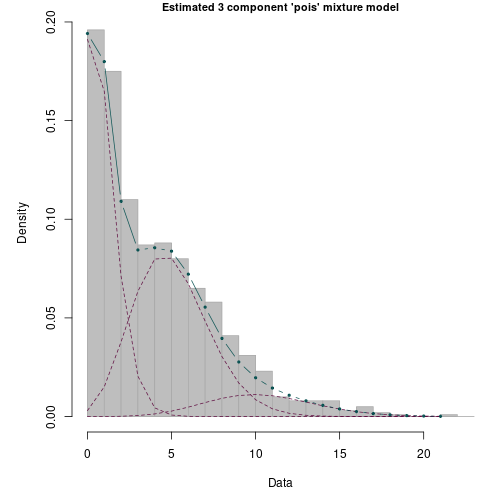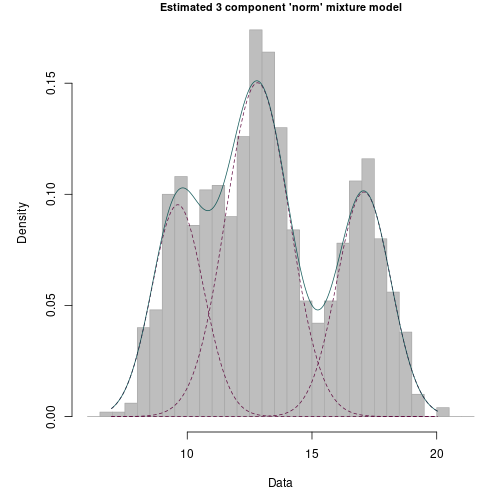For a real-world example, refer back to the faithful dataset and the corresponding datMix object which was created in Section 1. Fitting the distance methods to a continuous density requires a choice of bandwidth. While using the adaptive bandwidth is an option, if the user does not want to do so, it is recommended to use the function kdensity from the package kdensity [@kdensity] which automatically selects an optimal bandwidth. If the user wants to compare different bandwidth values, it is advisable to look at the plots of the respective kernel density estimates using kdensity and to choose one that captures the shape of the data well without fitting to noise. hellinger.cont fits a 2-component mixture to the data, which fits the data well and comprises similar parameter estimates to those found in the literature. # estimate the number of components: library(kdensity) res <- hellinger.cont(faithful.dM, bandwidth = kdensity(faithful.obs)$bw,
sample.n = 5000, threshold = "AIC")
#< Warning: While being used in Woo & Sriram's original paper, asymptotically, the
#< threshold 'AIC' does not go to 0 slower than the difference in squared Hellinger
#< distances once the correct order p is reached and the estimator is therefore not
#< consistent.
#<
#< Parameter estimation for a 1 component 'norm' mixture model:
#< Function value: 0.07305
#<                   w   mean     sd
#< Component 1:  1.000 70.749 13.298
#< Converged in 3 iterations.
#< ----------------------------------------------------------------------
#<
#< Parameter estimation for a 2 component 'norm' mixture model:
#< Function value: 0.001714
#<                     w     mean     sd
#< Component 1:  0.66051 79.38941 7.1255
#< Component 2:  0.33949 54.30404 6.5471
#< Converged in 3 iterations.
#< ----------------------------------------------------------------------
#<
#< Parameter estimation for a 3 component 'norm' mixture model:
#< Function value: 0.001687
#<                     w     mean     sd
#< Component 1:  0.16443 72.57078 6.8628
#< Component 2:  0.32200 53.81203 6.2690
#< Component 3:  0.51356 81.03060 6.4849
#< Converged in 3 iterations.
#< ----------------------------------------------------------------------
#<
#< The estimated order is 2.
plot(res)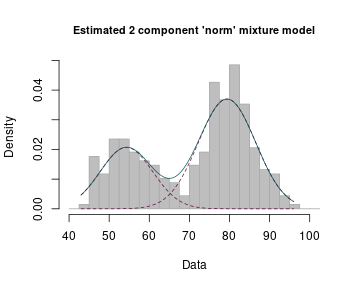At this point, it is worth having a closer look at the thresholds. They each satisfy $$t(j,n) \rightarrow 0$$ as $$n \rightarrow \infty$$, the sole condition the authors require. Now, the consistency proofs for the estimators defined in this Section all rely on the fact that, as $$n \rightarrow \infty$$, $D(\hat{f}_j, \tilde{f}_n) - D(\hat{f}_{j+1}, \tilde{f}_n) \rightarrow d_j > 0, \text{ for } j < p$ and $D(\hat{f}_j, \tilde{f}_n) - D(\hat{f}_{j+1}, \tilde{f}_n) \rightarrow 0, \text{ for } j \geq p,$ where $$p$$ is the true complexity (compare with [@l2, p. 4253, Proof of the Theorem], [@hell, p. 4383, Proof] and [@hellcont, p. 1485, Proof of Theorem 1]. If however $$t(j,n)$$ goes to $$0$$ faster than $$D(\hat{f}_j, \tilde{f}_n) - D(\hat{f}_{j+1}, \tilde{f}_n)$$ for $$j \geq p$$, asymptotically, the decision rule outlined above will always lead to $$j$$ being rejected. Therefore, a second condition should be placed on $$t(j,n)$$, namely choosing it in accordance with $D(\hat{f}_p, \tilde{f}_n) - D(\hat{f}_{p+1}, \tilde{f}_n) = o_p(t(j,n)).$

Both the LIC and the AIC as well as the SBC in the continuous case do not satisfy this condition, yet they are still part of the package for two reasons. First, since they were used in the original papers, they are included for the sake of completeness and reproducibility of original results. Second, consistency is an asymptotic property, and while the aforementioned thresholds do not fulfill it, they still perform well (and not rarely better than consistent thresholds) for smaller sample sizes. In the example above, the number of components is correctly identified under the non-consistent AIC threshold. Nonetheless, the user will get a warning when using one of non-consistent predefined thresholds.

The preceding example shows that $$\hat{p}$$ directly depends on the chosen threshold $$t(j, n)$$. While some thresholds can be motivated better than others from a theoretical perspective, the choice will ultimately always remain somewhat arbitrary. It would thus be desirable to have versions of the preceding functions which do not suffer from this drawback. L2.boot.disc, hellinger.boot.disc and hellinger.boot.cont all work similarly to their counterparts, with the exception that the difference in distances is not compared to a predefined threshold but a value generated by a bootstrap procedure. At every iteration (of $$j$$), the procedure sequentially tests $$p = j$$ versus $$p = j+1$$ for $$j = 1,2, \dots$$, using a parametric bootstrap to generate B samples of size $$n$$ from a $$j$$-component mixture given the previously calculated “best” parameter values $$(\hat{\mathbf{w}}^j, \hat{\mathbf{\theta}}^j)$$. For each of the bootstrap samples, again the “best” estimates corresponding to densities with $$j$$ and $$j+1$$ components are calculated, as well as their difference in distances from $$\tilde{f}_n$$. The null hypothesis $$H_0: p = j$$ is rejected and $$j$$ increased by $$1$$ if the original difference $$D(\hat{f}_j, \tilde{f}_n) - D(\hat{f}_{j+1}, \tilde{f}_n)$$ lies outside of the interval $$[ql, qu]$$, specified by the ql and qu empirical quantiles of the bootstrapped differences. Otherwise, $$j$$ is returned as the order estimate $$\hat{p}$$.

As an example, consider the poisson rMix object generated in the Section 2. It was a sample from the 3-component poisson mixture corresponding to $$\mathbf{w} = (0.45, 0.45, 0.1)$$ and $$\mathbf{\lambda} = (1, 5, 10)$$. The corresponding datMix object can be generated to use the function hellinger.boot.disc for identifying the number of components as follows:

poisList <- vector(mode = "list", length = 1)
names(poisList) <- "lambda"
poisList$lambda <- c(0, Inf) MLE.pois <- function(dat) mean(dat) pois.dM <- RtoDat(poisRMix, theta.bound.list = poisList, MLE.function = MLE.pois) set.seed(1) res <- hellinger.boot.disc(pois.dM, B = 50, ql = 0.025, qu = 0.975) plot(res)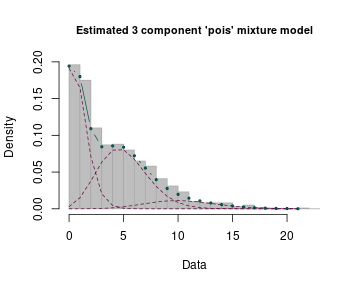## Section 5. Functions using LRTS As a third option of estimating the mixture complexity, the mixComp package provides an algorithm based on the likelihood ratio test statistic (LRTS), sequentially testing $$p = j$$ versus $$p = j+1$$ for $$j = 1,2, \dots$$, until the algorithm terminates. As noted in Section 1, it is not possible to use the generalized likelihood ratio test in the context of mixture models directly as the standard way of obtaining the asymptotic distribution of the LRTS under the null hypothesis cannot be applied. However, one can get estimates of the test's critical values by employing a bootstrap procedure. Making use of this approach, the function mix.lrt iteratively increases the assumed order $$j$$ and finds the MLE for both, the density of a mixture with $$j$$ and $$j+1$$ components, giving $$(\hat{\mathbf{w}}^{j}, \hat{\mathbf{\theta}}^{j}) \in W_j \times \Theta_j$$ and $$(\hat{\mathbf{w}}^{j+1}, \hat{\mathbf{\theta}}^{j+1}) \in W_{j+1} \times \Theta_{j+1}$$. It then calculates the corresponding LRTS, defined as $\text{LRTS}= -2\ln\left(\frac{L_0}{L_1}\right) \quad \text{, with}$ $L_0 = L_{\textbf{X}}(\hat{\mathbf{w}}^{j}, \hat{\mathbf{\theta}}^{j}) \quad\quad \text{and} \quad\quad L_1 = L_{\textbf{X}}(\hat{\mathbf{w}}^{j+1}, \hat{\mathbf{\theta}}^{j+1})\text{,}$ $$L_{\textbf{X}}$$ being the likelihood function given the data $${\textbf{X}}$$. Next, a parametric bootstrap is used to generate B samples of size $$n$$ from a $$j$$-component mixture given the previously calculated MLE $$(\hat{\mathbf{w}}^{j}, \hat{\mathbf{\theta}}^{j})$$. For each of the bootstrap samples, the MLEs corresponding to densities of mixtures with $$j$$ and $$j+1$$ components are calculated, as well as the LRTS. The null hypothesis $$H_0: p = j$$ is rejected and $$j$$ increased by $$1$$ if the LRTS based on the original data vector $$\textbf{X}$$ is larger than the chosen quantile of its bootstrapped counterparts. Otherwise, $$j$$ is returned as the order estimate $$\hat{p}$$. For further details, the reader is referred to [@lrt]. For example, refer back to the waiting variable from the faithful dataset. The mix.lrt function returns a $$2$$-component mixture with reasonable estimates for the component weights and parameters. set.seed(1) res <- mix.lrt(faithful.dM, B = 50, quantile = 0.95) print(res) plot(res)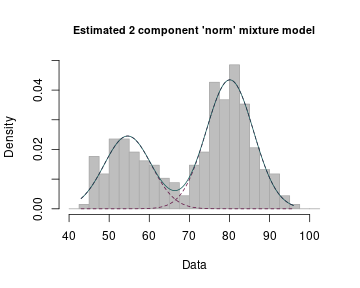Consider as another example the Acidity dataset (also included in the package) which comprises measurements of the acid neutralizing capacity (ANC) taken from 155 lakes in North-Central Wisconsin. The ANC indicates a lakes' capability to absorb acid, with low values potentially leading to a loss of biological resources. This dataset has been first analysed as a mixture of normal distributions on the log scale by [@acidity]. The paper suggests the number of components to equal 2 (with 3 also being considered). The mix.lrt method agrees with such conclusions, returning a 2-component mixture with reasonable estimates for the component weights and parameters. acidity.obs <- unlist(acidity) acidity.dM <- datMix(acidity.obs, dist = "norm", discrete = FALSE, MLE.function = MLE.norm.list, theta.bound.list = norm.bound.list) set.seed(0) res <- mix.lrt(acidity.dM, B = 50, quantile = 0.95) plot(res)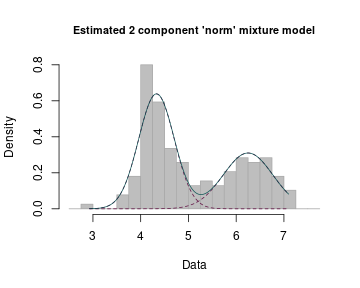## Section 6. Non-standard mixtures In all preceding examples, the families of component densities $$\{g(x;\theta):\theta \in \Theta \}$$ belonged to one of the “standard” probability distributions included in the stats package, which provides the density/mass function, cumulative distribution function, quantile function and random variate generation for selected distributions. The function names are of the form dxxx, pxxx, qxxx and rxxx respectively. With some additional effort, it is possible to use the mixComp package on “non-standard” distributions – the user merely has to provide functions evaluating the density and generating random numbers for the component distribution. In accordance with R conventions, the user-generated function dxxx has to take x and the distribution parameters as input and returns the value of the density function specified by the parameters at the point x. Likewise, rxxx requires n and the distribution parameters as input and returns n random numbers based on the distribution specified by the aforementioned parameters. As an example, consider a sample $$\textbf{X}$$ from a 3-component mixture of normals with means equal to $$10, 11$$ and $$13$$. Assume that the standard deviation of all components is known to be $$0.5$$, yet the number of components and their means are unknown. Then each of the components follows a $$\mathcal{N}(\mu, 0.5)$$ distribution, which shall be called norm0.5. The first step is always that of creating the dxxx and rxxx functions, since they will be called by the mixComp functions. The following example creates the Mix and rMix objects based on the density of a normal mixture with $$\mathbf{w} = (0.3, 0.4, 0.3)$$, $$\mathbf{\mu} = (10, 11, 13)$$ and $$\mathbf{\sigma} = (0.5, 0.5, 0.5)$$ and plots the obtained mixture density and the corresponding random sample. dnorm0.5 <- function(x, mean){ dnorm(x, mean = mean, sd = 0.5) } rnorm0.5 <- function(n, mean){ rnorm(n, mean = mean, sd = 0.5) } ## create objects Mix and rMix: set.seed(1) norm0.5Mix <- Mix("norm0.5", discrete = FALSE, w = c(0.3, 0.4, 0.3), mean = c(10, 11, 13)) norm0.5RMix <- rMix(1000, obj = norm0.5Mix) ## plot the results: plot(norm0.5Mix) plot(norm0.5RMix)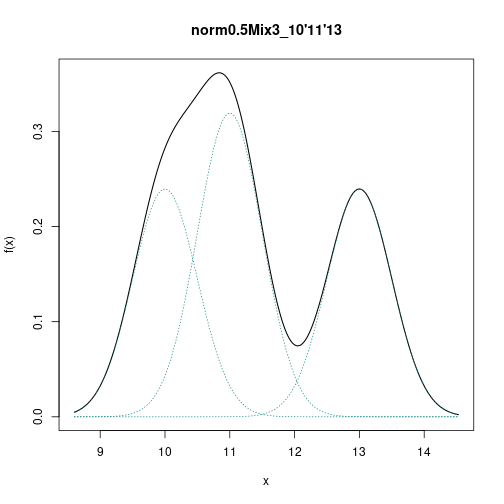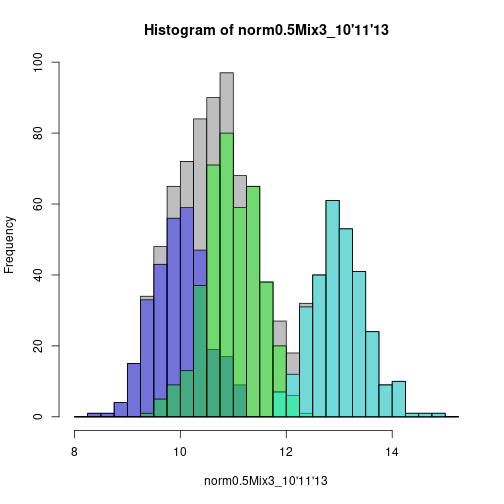Below we will estimate of the mixture density using mix.lrt given a sample from the 3-component normal mixture with $$\mathbf{w} = (0.3, 0.4, 0.3)$$, $$\mathbf{\mu} = (10, 11, 13)$$, $$\mathbf{\sigma} = (0.5, 0.5, 0.5)$$. We start by creating all necessary inputs: norm0.5.list <- vector(mode = "list", length = 1) names(norm0.5.list) <- c("mean") norm0.5.list$mean <- c(-Inf, Inf)

MLE.norm0.5 <- function(dat) mean(dat)

norm0.5.dM <- RtoDat(norm0.5RMix, theta.bound.list = norm0.5.list,
MLE.function = MLE.norm0.5)


Finally, the mixComp procedures can be used on the datMix object as usual. The results can be printed and plotted using print and plot functions.

set.seed(1)
res <- mix.lrt(norm0.5.dM, B = 50, quantile = 0.95)

print(res)
#<
#< Parameter estimation for a 3 component 'norm0.5' mixture model:
#< Function value: 1546.6176
#<                    w    mean
#< Component 1: 0.39955 11.0148
#< Component 2: 0.28695 13.0085
#< Component 3: 0.31350  9.9905
#< Converged in 3 iterations.
#<
#< The estimated order is 3.
plot(res)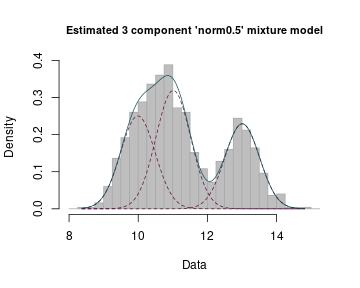## Section 7. Computational nuance for mixComp functions using the solnp() solver

Several functions in the mixComp package (namely, nonparamHankel, paramHankel, hellinger.cont, hellinger.disc, L2.disc, mix.lrt) make use of solnp() function (Rsolnp library), which is a solver for general nonlinear programming problems. The above mentioned mixComp functions attempt to generate good starting values by clustering the data via clara function prior to applying solnp(), which lead to convergence of the algorithm in most of the cases. However when running multiple simulations, solnp() might not converge for particular initial values with default control values. This may happen when very few observations are assigned to some of the clusters have, in which case the solver can get “stuck”, not even resulting in bad exit status (codes 1 and 2 in returned by solnp() convergence value). This issue can be overcome by specifying the control parameters in the functions using the solnp() solver (e.g. by defining L2.boot.disc(geom.dM, j.max = 5, B = 500, ql = 0.025, qu = 0.975, control = list("rho" = 0.1, tol = 0.0000001, trace = 0)), see description of the solnp() function for further details on setting the control parameters) or by setting a time limit for the function execution and going to the next iteration whenever the time limit is exceeded.

## Section 8. Summary and discussion

In this paper we presented the R package mixComp, a collection of routines developed to estimate a mixture's complexity from a data sample $$\textbf{X}$$. Moreover, it provides the possibility of generating artificial data from specified mixtures as well as proper visualization tools for the complexity estimation result, plotting either the successive determinant values or the final fitted mixture. If estimates of the component weights and parameters are sought, $$\hat{p}$$ can be passed to one of the many R packages specialized in their calculation, or one of the mixComp functions returning weight and parameter estimates can be used. However, it should be noted that the theory on which this package is based concentrates on showing consistency for $$\hat{p}$$ – other estimates obtained in the process are merely by-products. A primary goal of the package was to make it extendible, meaning that it can be utilized on mixtures of less well-known distributions, as long as sufficient information on the density and random variate generation is provided. We hope that this package will be useful for practitioners in the many areas where mixture models are applicable.

## Computational details

All computations and graphics in this paper have been done using R version 4.0.0 with the packages boot 1.3-24, cluster 2.1.0, expm 0.999-4, matrixcalc 1.0-3, Rsolnp 1.16 and kdensity 1.0.1. R itself and all packages used are available from the Comprehensive R Archive Network (CRAN) at https://CRAN.R-project.org/.

## Appendix

### Distance and non-parametric estimator definitions

Let $$\textbf{X} = \{X_1, \dots, X_n\}$$ be an i.i.d. sample of size $$n$$ from some continuous distribution with unknown density $$f$$. Its kernel densty estimator is defined as $\tilde{f}_n(x):= \frac{1}{nc_n}\sum_{i=1}^n K\left( \frac{x-X_i}{c_n} \right),$ with kernel $$K$$ and bandwidth $$c_n$$.

$\tilde{f}_n(x) := \frac{1}{n}\sum_{i=1}^n \sum_{j=1}^k \frac{a_j(X_i)}{c_{n, j}} K\left( \frac{x-X_i}{c_{n, j}} \right),$

with kernel $$K$$, bandwidth $$c_{n, j}$$ and weights (positive and summing to $$1$$) $$a_j(X_i)$$.

Let $$\textbf{X} = \{X_1, \dots, X_n\}$$ be an i.i.d. sample of size $$n$$ from some discrete distribution with unknown probability mass function $$f$$. Its empirical probability mass function is defined as $\tilde{f}_n(x) := \frac{1}{n}\sum_{i=1}^n \mathbf{1}_{\{X_i = x\}}.$

Let $$g, f$$ be two probability mass functions defined on $$\mathcal{X}$$. The squared $$L_2$$ distance between $$g$$ and $$f$$ is given by $L_2^2(g,f) := \sum_{\mathcal{X}} (g(x)-f(x))^2.$

Let $$g, f$$ be two density or probability mass functions defined on $$\mathcal{X}$$. The squared Hellinger distance between $$g$$ and $$f$$ is given by $H^2(g,f) := \sum_{\mathcal{X}} \left( \sqrt{g(x)}-\sqrt{f(x)} \right)^2$ in the discrete case and by $H^2(g,f) := \int_{\mathcal{X}} \left( \sqrt{g(x)}-\sqrt{f(x)} \right)^2 dx$ in the continuous case.

### Further results on the real-world datasets

The Table below showcases the results of all complexity estimation procedures when applied to the four real-world datasets that were discussed in this paper. The table also includes the results for the Shakespeare dataset borrowed from [@shakespeare]. It was not mentioned in the vignette but is included in the mixComp package. The following settings were used to calculate these results (default setting were used unless indicated otherwise):

• set.seed(1) was used for all complexity calculations,
• for hellinger.cont and hellinger.boot.cont the bandwidths suggested by kdensity were used,
• for nonparamHankel, we used $$A(j)l(n) = \frac{j\log(n)}{\sqrt{n}}$$ and j.max = 6,
• for the bootstrapped distance and the LRTS methods, we used B = 50.

#### Table A: Results for all mixComp methods used on real-world data

Method Children Old Faithful Shakespeare Acidity
nonparamHankel 2 x 2 x
nonparamHankel(scaled) 2 x 2 x
paramHankel 2 x 2 x
paramHankel.scaled 2 x 2 x
L2.disc 2 x 3 x
L2.boot.disc 2 x 4 x
hellinger.disc 2 x 3 x
hellinger.boot.disc 2 x 3 x
hellinger.cont x 2 x 2
hellinger.boot.cont x 2 x 2
mix.lrt 2 2 3 2

\pagebreak